# SAT II Math I : Elementary Operations

## Example Questions

### Example Question #20 : How To Multiply Integers

Evaluate the expression.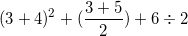Possible Answers: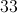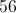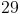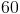Correct answer:Explanation:

Follow the correct order of operations: parenthenses, exponents, multiplication, division, addition, subtraction.First, evaluate any terms in parenthesis.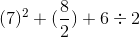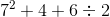Next, evaluate the exponent.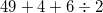Divide.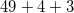Finally, add.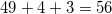### Example Question #1 : Elementary Operations

Evalute the expression: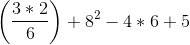Possible Answers: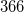Correct answer:Explanation:

Follow the correct order of operations: parentheses, exponents, multiplication, division, addition, subtraction. (This is typically abbreviated as PEMDAS. Note that both multiplication and division, and addition and subtraction, are equal to each other in terms of rank, so when both are present, solving the equation proceeds from left to right).

First, simplify anything in parentheses.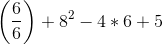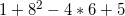Next, simplify any terms with exponents.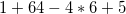Now, perform multiplication.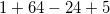Since all we are left with is addition and subtraction, we perform simplification from left to right.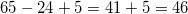Thus, our answer is:### Example Question #2 : Mathematical Relationships

Add in modulo 7: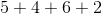Possible Answers:Correct answer:Explanation:

In modulo 7 arithmetic, a number is congruent to the remainder of its division by 7.

Therefore, since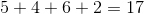and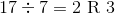,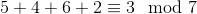,

and the correct response is 3.

### Example Question #1 : Mathematical Relationships

Add: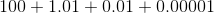Possible Answers: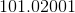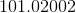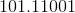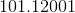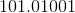Correct answer:Explanation:

To solve, make sure the digits are aligned with the correct placeholder.  It is also possible to add term by term.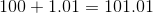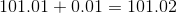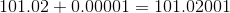The correct answer is:### Example Question #4 : Mathematical Relationships

Evaluate: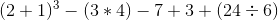.

Possible Answers:Correct answer:Explanation:

Step 1: Recall PEMDAS...

Parentheses, Exponents, Multiplication, Division, Addition, Subtraction.

Step 2: Perform the evaluation in separate pieces...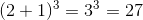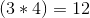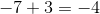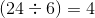Step 3: Replace the values and keep the signs..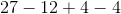Step 4: Evaluate: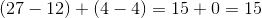### Example Question #5 : Mathematical Relationships

Find the sum of the numbers: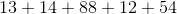Possible Answers: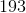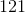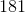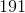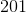Correct answer:Explanation:

Add all the ones digits.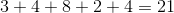Add the tens digits with the two as the carryover.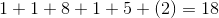Combine this value with the ones digit of the first number.

The answer is:### Example Question #6 : Mathematical Relationships

Evaluate: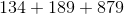Possible Answers: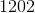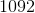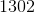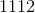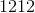Correct answer:Explanation:

Add the ones digits.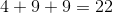Add the tens digits with the tens digit of the previous number as carryover.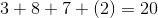Repeat the process with the hundreds digits.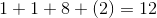Combine this number with the ones digits of the previous calculations.

The answer is:### All SAT II Math I Resources